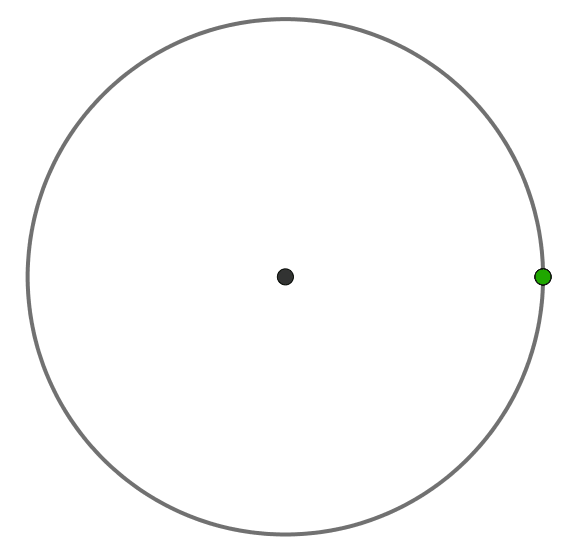# Round and round they go

Calculus Level 3

Three points ${\color{#3D99F6}A}, {\color{#D61F06}B},$ and ${\color{#20A900}C}$ are moving counterclockwise around a unit circle with periods $\SI{1}{\second}, \SI{2}{\second},$ and $\SI{3}{\second},$ respectively.

They started from the same position on the circle.

What is the maximal area of triangle ${\color{#3D99F6}A}{\color{#D61F06}B}{\color{#20A900}C}?$×